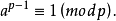# 伪素数

Fermat’s theorem states that for any prime number p and for any integer a > 1, a^p == a (mod p). That is, if we raise a to the pth power and divide by p, the remainder is a. Some (but not very many) non-prime values of p, known as base-a pseudoprimes, have this property for some a. (And some, known as Carmichael Numbers, are base-a pseudoprimes for all a.)

Given 2 < p ≤ 1,000,000,000 and 1 < a < p, determine whether or not p is a base-a pseudoprime.
Input
Input contains several test cases followed by a line containing “0 0”. Each test case consists of a line containing p and a.
Output
For each test case, output “yes” if p is a base-a pseudoprime; otherwise output “no”.
Sample Input
3 2
10 3
341 2
341 3
1105 2
1105 3
0 0

Sample output
no
yes
no
yes
yes

（费马小定理＋快速幂）#include<iostream>
#include<cmath>
using namespace std;
bool is_prime(long long n)
{
if(n==0||n==1)return false;
for(long long i=2; i<=sqrt(n+0.5); i++)
if(n%i==0)
return false ;
return true;
}
long long quick_pow(long long a,long long b,long long c)
{
long long res=1;
while(b)
{
if(b&1)
res=res*a%c;
a=(a*a)%c;
b>>=1;
}
return res;
}
int main()
{
long long a,p;
while(cin>>p>>a)
{
if(p==0&&a==0)
break;
if(is_prime(p))
{
cout<<"no"<<endl;
}else {
if(quick_pow(a,p,p)==a)
{
cout<<"yes"<<endl;
}else {
cout<<"no"<<endl;
}
}
}
}



©️2019 CSDN 皮肤主题: 大白 设计师: CSDN官方博客